Pascal's Law, Buoyancy and Archimedes Principle (Old Syllabus)

# Pascal's Law, Buoyancy and Archimedes Principle (Old Syllabus) - Science Class 9

## Pressure in Fluids

A substance which can flow is called a fluid. All liquids and gases are thus fluids. We know that a solid exerts pressure on a surface due to its weight. Similarly, a fluid exerts pressure on the container in which it is contained due to its weight. However, unlike a solid, a fluid exerts pressure in all directions.
A fluid contained in a vessel exerts pressure at all points of the vessel and in all directions.
All the streams of water reach almost the same distance in the air.

### Pascal's Law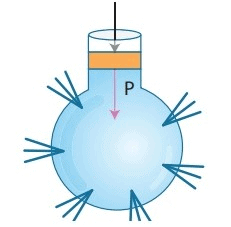Pascal's LawIn an enclosed fluid, if pressure is changed in any part of the fluid, then this change of pressure is transmitted undiminished to all the other parts of the fluid.

Question for Pascal's Law, Buoyancy and Archimedes Principle (Old Syllabus)
Try yourself:
Which of the following statements is true regarding the pressure exerted by a fluid?

## Buoyancy

When a body is partially or wholly immersed in a liquid, an upward force acts on it which is called upthrust or buoyant force. The property of the liquids responsible for this force is called buoyancy.

### Buoyancy is a Familiar Phenomenon

A body immersed in water seems to weigh less than when it is in air. When the body is less dense than the fluid, then it floats. The human body usually floats in water, and a helium filled balloon floats in air.

When a body is immersed in a fluid (liquid or gas) it exerts an upward force on the body. This upward force is called upthrust or buoyant force (U or FB) and the phenomenon is termed as buoyancy. Thus buoyancy or upthrust is the upward force exerted by a fluid (liquid or gas) when a body is immersed in it.

It is a common experience that when a piece of cork is placed in water it floats with two-fifth of its volume inside water. If the cork piece is pushed into water and released it comes to the surface as if it has been pushed by someone from inside due to the buoyant force exerted by fluid.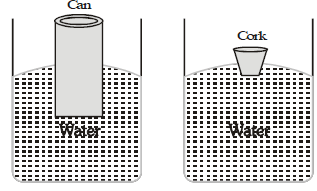### Factor on which Buoyancy Depends

Upthrust depends upon the following two factors :

(a) The volume of the body submerged in the fluid. :- It is found that greater the volume of a body greater is the upthrust it experience when inside a fluid.
(b) The density of fluid in which the body is immersed :- It is also found that greater the density of the fluid greater is the upthrust it applies on the body.

### Effect of Buoyancy

When a body is partially or fully immersed in a fluid, then following vertical forces are experienced by it.
(a) Its weight (W) acting vertically downwards through the centre of the body.
(b) Force of buoyancy (U or B or FB) or upthrust, acting vertically upwards through the centre of gravity of the body.
The following three cases arise:

(1) When W < U, the body floats: In this case the body will rise above the surface on the liquid to the extent that the weight of the liquid displaced by its immersed part equals the weight of the body. Then the body will float with only a part of it immersed in the liquid. In this case Vrg < Vsg or r < s. Thus if a cork, which has a density less than that of water will rise in water till a portion of it is above water. Similarly a ship floats in water since its density is less than the density of water.

(2) When W > U, the body sinks: If r and s present the densities of the body and the fluid respectively and V the volume of the body (which is also the volume of the fluid displaced) then Vrg > Vsg or r > s i.e., the body sinks in the fluid in case its density is greater than the density of the fluid. An iron nail has greater density than water, therefore it sinks in water.

(3) When W = U: The resultant force acting on the body when fully immersed in the fluid is zero. The body is at rest anywhere within the fluid. The apparent weight of the body is zero for all such positions.
Thus, we find that a body will float when its weight is equal to the weight U of the fluid displaced i.e. the upthrust.

## Archimedes Principle

Archimedes principle states that: "Anybody completely or partially submerged in a fluid is buoyant up by a force equal to the weight of the fluid displaced by the liquid".

In other words: "When a body is partially or completely immersed in a fluid, the fluid exerts an upward force on the body equal to the weight of the fluid displaced by the body.

### Experimental Verification of Archimedes Principle

Consider a container C1 filled with water up to the level from where pipe P extends out. The other end of pipe P opens to a small container C2 placed on a weighing balance which measure 00.00 [after the placement of the container C2]. A block B hangs on a spring balance S which shows a reading of 7 kg.

(a) If we partially immerse the block in water we observe some water flows out from C1 to C2 through P. The weighing machine shows a reading 1 kg and the loss of reading in spring balance is 7 - 6 = 1kg. This means that weight of water displaced by the block is equal to loss in weight of block.

(b) Now we completely immerse the block in water, we observe that the weight of water displaced by the block is 5 kg and the reading in spring balance is 2 kg. The loss of weight of block is 7 - 2 = 5 kg. Again we reach the same conclusion that weight of water displaced by the block is equal to the loss in weight of block.

(c) What happens when the block is further immersed? No more water will be displaced by the block and therefore reading shown by weighing machine and spring balance remains unchanged.

Why the spring balance shows a loss in weight of the block when Fig. A block of 7 kg hanging on a the block is immersed in water? This is because of buoyant force on spring balance acting vertically upwards. The loss in weight is equal to the buoyant force.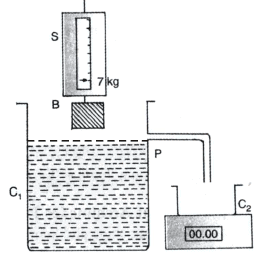Conclusion 1:

Buoyant force ∝ volume of liquid displaced (V).

If two bodies of different material have same volume, the buoyant force acting on them, when completely immersed in water, is same.
Instead of water if we take a liquid lighter than water then the volume displaced by the block on complete immersion will be the same but the buoyant force will be less. This is because the density of lighter liquid is less than that of water.
We know that Density =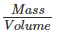Mass = Density x Volume
For lighter liquids, the mass of the liquid displaced is less even when the volume displaced is the same.

Conclusion 2:
Buoyant force ∝ Density of liquid
i.e., Buoyant force ∝ d
It has also been found that buoyant force also depends on the acceleration due to gravity.

Conclusion 3:
Buoyant force ∝ g
If we combine all the three, we get
Buoyant force ∝ V dg
⇒ Buoyant force ∝ mg [mass = V x d]
⇒ Buoyant force ∝ Weight of the liquid displaced

Note: A body placed in a gaseous medium is also acted by the upthrust equal to the weight of the gas displaced.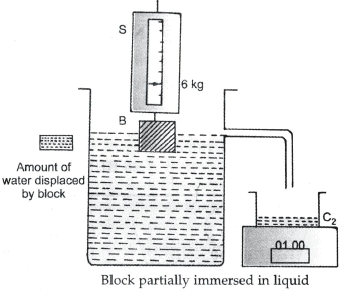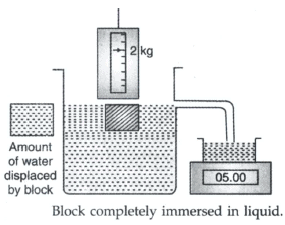The above facts has been summarised in Archimedes principle which states that the upward force acting on a solid body which is partially or completely immersed in a fluid, is equal to the weight of the fluid displaced.

This upward force is called buoyant force or upthrust.

### Applications of Archimedes Principle

1. In designing ships and submarines.

2. Lactometer is based on the Archimedes principle. It is used to determine the purity of a sample of milk.

3. Hydrometer is also based on the Archimedes principle. It is used to determine the density of liquids.

Question for Pascal's Law, Buoyancy and Archimedes Principle (Old Syllabus)
Try yourself:
A body is partially immersed in a liquid. What vertical forces does the body experience?

(a) Ships:
Although it is made of iron and steel which are materials denser than water a ship floats in water. This is due to the fact that a floating ship displaces a weight of water equal to its own weight including that of the cargo. The volume of the ship is much larger than the volume of the material with which it is made. Since the empty space in the ship contains air therefore its average density is less than the density of water. Thus a ship floats with a small section under water.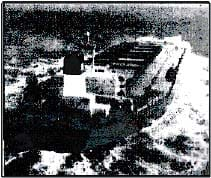A ship has to move in waters of different seas which have different densities. As a result it sinks more in water with less density than in water with more density. Therefore ships are marked with white lines on its sides called load lines of pimsoll marks. The load lines (called the Plimsoll mark) on the side of a ship of a ship show how low in the water it can lie and still be safely and legally loaded under different conditions.

(b) Submarines:
A submarine, figure sinks by taking water into its buoyancy tanks. Once submeged, the upthrust is unchanged but the weight of the submarine increases with the inflow of water and it sinks faster. To surface, compressed air is used to blow the water out of the tanks.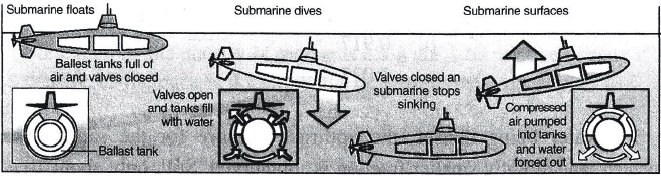Each submarine is provided with ballast tanks. If the submarine has to submerge these tanks are filled with water. This makes the average density of the submarine greater than that of water as a result it sinks. When the submarine has to be surfaced, compressed air is blown into these tanks to expel the water. Again the average density of the submarine becomes less than that of water, hence it floats.

The document Pascal's Law, Buoyancy and Archimedes Principle (Old Syllabus) | Science Class 9 is a part of the Class 9 Course Science Class 9.
All you need of Class 9 at this link: Class 9

## Science Class 9

66 videos|355 docs|97 tests

## FAQs on Pascal's Law, Buoyancy and Archimedes Principle (Old Syllabus) - Science Class 9

 1. What is the definition of pressure in fluids?Ans. Pressure in fluids refers to the force exerted by the fluid per unit area. It is the result of the fluid particles colliding with the walls of the container or any other surface they come into contact with.
 2. How does Pascal's law relate to pressure in fluids?Ans. Pascal's law states that the pressure applied to a fluid enclosed in a container is transmitted equally in all directions. This means that any change in pressure at one point in a fluid will be transmitted to all other points in the fluid.
 3. What is buoyancy?Ans. Buoyancy is the upward force exerted on an object submerged in a fluid, either partially or completely. This force is equal to the weight of the fluid displaced by the object and is responsible for making objects float or sink in fluids.
 4. How does Archimedes' principle explain buoyancy?Ans. Archimedes' principle states that when an object is partially or fully submerged in a fluid, it experiences an upward buoyant force equal to the weight of the fluid it displaces. If the buoyant force is greater than the weight of the object, it will float; if it is less, the object will sink.
 5. How can we calculate the buoyant force acting on an object?Ans. The buoyant force can be calculated using Archimedes' principle. It is equal to the weight of the fluid displaced by the object. The formula is: Buoyant force = weight of the fluid displaced = density of the fluid x volume of the fluid displaced x acceleration due to gravity.

## Science Class 9

66 videos|355 docs|97 testsExplore Courses for Class 9 examSignup to see your scores go up within 7 days! Learn & Practice with 1000+ FREE Notes, Videos & Tests.
10M+ students study on EduRev
Track your progress, build streaks, highlight & save important lessons and more!
Related Searches

,

,

,

,

,

,

,

,

,

,

,

,

,

,

,

,

,

,

,

,

,

,

,

,

;